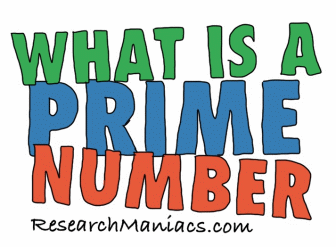What is a prime number?You are getting old and past your prime when you realize that you do not remember the definition of a prime number. You ask yourself: What is a prime number?

You have come to the right place for all your prime number facts.

First of all, a prime number must be an integer. Integer means that it must be a whole number. A prime number cannot have decimal points.

Secondly, a prime number must be a positive number. Thus, a prime number cannot be a negative number.

Thirdly, a prime number must be greater than 1. Thus, 1 itself is not a prime number.

Fourth, a prime number can only be divisible by 2 positive integers. One of the integers must be 1 and the other integer must be the prime number itself.

Fifth, All prime numbers are odd numbers except the number 2.

Here are a few examples to illustrate better what a prime number is:

Is -5 a prime number?
No, a negative number cannot be a prime number.

Is 1 a prime number?
No, 1 is not a prime number because it is not greater than 1.

Is 2 a prime number?
Yes, 2 is a whole positive number that is only divisible by itself and 1. 2 is also the only exception to the rule that a prime number must be odd.

Is 5.2 a prime number?
No, a prime number must be a whole number.

Is 28 a prime number?
No, 28 is not a prime number because it is divisible by more than 1 and itself. It is also divisible by 2, 4, and 7.

Is 29 a prime number?
Yes, 29 is a positive whole odd number greater than 1 that is only divisible by 1 and itself.

Here is a list of all prime numbers up to 1000:

2 3 5 7 11 13 17 19 23 29 31 37 41 43 47 53 59 61 67 71 73 79 83 89 97 101 103 107 109 113 127 131 137 139 149 151 157 163 167 173 179 181 191 193 197 199 211 223 227 229 233 239 241 251 257 263 269 271 277 281 283 293 307 311 313 317 331 337 347 349 353 359 367 373 379 383 389 397 401 409 419 421 431 433 439 443 449 457 461 463 467 479 487 491 499 503 509 521 523 541 547 557 563 569 571 577 587 593 599 601 607 613 617 619 631 641 643 647 653 659 661 673 677 683 691 701 709 719 727 733 739 743 751 757 761 769 773 787 797 809 811 821 823 827 829 839 853 857 859 863 877 881 883 887 907 911 919 929 937 941 947 953 967 971 977 983 991 997

You can also enter a number below to see if it is a prime number.